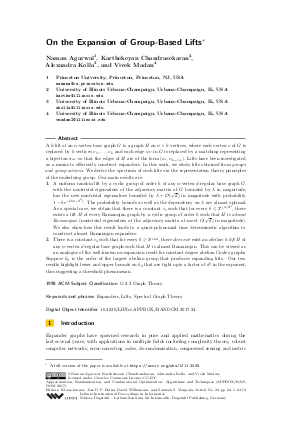Document# On the Expansion of Group-Based Lifts

### Authors Naman Agarwal, Karthekeyan Chandrasekaran, Alexandra Kolla, Vivek Madan## File

LIPIcs.APPROX-RANDOM.2017.24.pdf
• Filesize: 0.5 MB
• 13 pages

## Cite As

Naman Agarwal, Karthekeyan Chandrasekaran, Alexandra Kolla, and Vivek Madan. On the Expansion of Group-Based Lifts. In Approximation, Randomization, and Combinatorial Optimization. Algorithms and Techniques (APPROX/RANDOM 2017). Leibniz International Proceedings in Informatics (LIPIcs), Volume 81, pp. 24:1-24:13, Schloss Dagstuhl - Leibniz-Zentrum für Informatik (2017)
https://doi.org/10.4230/LIPIcs.APPROX-RANDOM.2017.24

## Abstract

A k-lift of an n-vertex base graph G is a graph H on nxk vertices, where each vertex v of G is replaced by k vertices v_1,...,v_k and each edge uv in G is replaced by a matching representing a bijection pi_{uv} so that the edges of H are of the form (u_i,v_{pi_{uv}(i)}). Lifts have been investigated as a means to efficiently construct expanders. In this work, we study lifts obtained from groups and group actions. We derive the spectrum of such lifts via the representation theory principles of the underlying group. Our main results are: 1. A uniform random lift by a cyclic group of order k of any n-vertex d-regular base graph G, with the nontrivial eigenvalues of the adjacency matrix of G bounded by lambda in magnitude, has the new nontrivial eigenvalues bounded by lambda+O(sqrt{d}) in magnitude with probability 1-ke^{-Omega(n/d^2)}. The probability bounds as well as the dependency on lambda are almost optimal. As a special case, we obtain that there is a constant c_1 such that for every k<=2^{c_1n/d^2}, there exists a lift H of every Ramanujan graph by a cyclic group of order k such that H is almost Ramanujan (nontrivial eigenvalues of the adjacency matrix at most O(sqrt{d}) in magnitude). We also show how this result leads to a quasi-polynomial time deterministic algorithm to construct almost Ramanujan expanders. 2. There is a constant c_2 such that for every k>=2^{c_2nd}, there does not exist an abelian k-lift H of any n-vertex d-regular base graph such that H is almost Ramanujan. This can be viewed as an analogue of the well-known no-expansion result for constant degree abelian Cayley graphs. Suppose k_0 is the order of the largest abelian group that produces expanding lifts. Our two results highlight lower and upper bounds on k_0 that are tight upto a factor of d^3 in the exponent, thus suggesting a threshold phenomenon.
##### Keywords
• Expanders
• Lifts
• Spectral Graph Theory

## Metrics

• Access Statistics
• Total Accesses (updated on a weekly basis)
0

## References

1. L. Addario-Berry and S. Griffiths. The spectrum of random lifts. Preprint arXiv:1012.4097, 2010.2. N. Agarwal, K. Chandrasekaran, A. Kolla, and V. Madan. On the expansion of group-based lifts. Preprint arXiv:1311.3268, 2016. URL: https://arxiv.org/abs/1311.3268.
3. Y. Bilu and N. Linial. Lifts, discrepancy and nearly optimal spectral gap. Combinatorica, 26(5):495-519, 2006.4. C. Bordenave. A new proof of friedman’s second eigenvalue theorem and its extension to random lifts. Preprint arXiv:1502.04482, 2015.5. K. Chandrasekaran and A. Velingker. Shift lifts preserving ramanujan property. Linear Algebra and its Applications, 529:199-214, 2017.6. F. Chung. Diameters and eigenvalues. Journal of the American Mathematical Society, 2(2):187-196, 1989.7. M. Cohen. Ramanujan graphs in polynomial time. In 2016 IEEE 57th Annual Symposium on Foundations of Computer Science (FOCS), pages 276-281, 2016.8. R. Feng, J. Kwak, and J. Lee. Characteristic polynomials of graph coverings. Bull. Austal. Math. Soc., 69:133-136, 2004.9. J. Friedman. Relative expanders or weakly relatively ramanujan graphs. Duke Math. J, 118:2003, 2003.10. J. Friedman. A proof of alon’s second eiganvalue conjecture and related problems. Mem. Amer. Math,Soc, 195(910), 2008.11. J. Friedman, J. Kahn, and E. Szemerédi. On the second eigenvalue of random regular graphs. In Proceedings of the Twenty-first Annual ACM Symposium on Theory of Computing, STOC'89, pages 587-598, 1989.12. J. Friedman and D.-E. Kohler. The Relativized Second Eigenvalue Conjecture of Alon. Preprint arXiv:1403.3462, 2014.13. J. Friedman, R. Murty, and J. Tillich. Spectral estimates for abelian cayley graphs. J. Comb. Theory Ser. B, 96(1):111-121, 2006.14. Y. Greenberg. On the spectrum of graphs and their universal coverings. Ph.D Thesis, 1995.15. Chris Hall, Doron Puder, and William F. Sawin. Ramanujan coverings of graphs. In Proceedings of the Forty-eighth Annual ACM Symposium on Theory of Computing, STOC'16, pages 533-541, 2016.16. S. Hoory, N. Linial, and A. Wigderson. Expander graphs and their applications. Bull. Amer. Math. Soc, 43(4):439-561, 2006.17. N. Linial and D. Puder. Word maps and spectra of random graph lifts. Random Struct. Algorithms, 37(1)):100-135, 2010.18. E. Lubetzky, B. Sudakov, and V. Vu. Spectra of lifted ramanujan graphs. Advances in Mathematics, 227:1612–1645, 2011.19. A. Lubotzky, R. Phillips, and P. Sarnak. Ramanujan graphs. Combinatorica, 8(3):261-277, 1988.20. A. Makelov. Expansion in lifts of graphs, 2015. Undergraduate Thesis, Harvard University.21. A. Marcus, D. Spielman, and N. Srivastava. Interlacing families i: Ramanujan graphs of all degrees. In Proceedings, FOCS 2013, 2013.22. A. Marcus, D. Spielman, and N. Srivastava. Interlacing families iv: Bipartite ramanujan graphs of all sizes. In IEEE 56th Annual Symposium on Foundations of Computer Science, pages 1358-1377, 2015.23. G. Margulis. Explicit group-theoretic constructions of combinatorial schemes and their applications in the construction of expanders and concentrators. Probl. Inf. Transm, 24(1):39-46, 1988.24. H. Mizuno and I. Sato. Characteristic polynomials of some graph coverings. Discrete Mathematics, 142:295-298, 1995.25. A. Nilli. On the second eigenvalue of a graph. Discrete Math, 91(2):207-210, 1991.26. M. Pinsker. On the complexity of a concentrator. 7th International Teletraffic Conference, pages 318/1-318/4, 1973.27. D. Puder. Expansion of random graphs: New proofs, new results. Preprint arXiv:1212.5216, 2013.28. P. Sarnak. What is an expander? Notices Amer. Math. Soc, 51(7):762-763, 2006.X

Feedback for Dagstuhl Publishing